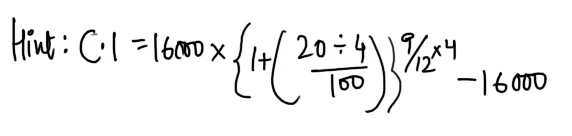New Student User - Use Code HELLO

# Compound Interest : 10 Questions Repeated in the Exams

#### Ques 1.

In how many years will a sum of Rs 800 at 10% per annum compound interest .The rate % is
(a) 1 1/2
(b) 2 1/2
(c) 1 1/3
(d) 4 1/4
Ans 1.  1  1/2

#### Ques 2.

What is the amount payable after two Years, if it is put at 20% p.a. compound interest of 5 paise per rupee per annum, compounded annually ?
(a) Rs 10025
(b) Rs 12000
(c) Rs 11025
(d) Rs 11125
Ans 2. 11025[ x paise  per rupee p.a. = x % p.a.]

#### Ques 3.

The compound interest on Rs 16000 at 20% p.a. for 9 months, Compounded quarterly is
(a) Rs 2225
(b) Rs 2425
(c) Rs 2012
(d) Rs 2522
Ans 3. Rs 2522#### Ques 4.

A sum of money becomes p 4 times itself in 2 years at compound interest .The rate of Interest is
(a) 75%
(b) 100%
(c) 50%
(d) Indeterminate
Ans 5. 100%

#### Ques 5.

An amount deposited on compound interest becomes Rs 5700 after three years and Rs 6000 after four years. After 5 Years this amount will become
(A) Rs 6308
(b) Rs 6816
(c) Rs 6410
(d) Rs 6316
Ans 5. 6316#### Ques 6.

Every year an amount increase by 1/8th of itself .How much will it be after two years if its present value is Rs 64000 ?
(a) Rs 81000
(b) Rs 80000
(c) Rs 75000
(d) None of these
Ans 6.  Rs 81000
Hint : Rate % = 1/8 x 100% = 12 .5 %

#### Ques 7.

A sum of money lent at compound interest amounts to Rs 6050.00 in two years and to Rs 7320.50 in four years. The rate of interest is
(a) 12%
(b) 5 %
(c) 10%
(d) 8 %
Ans 7.   10%

#### Ques 8.

A sum of money at compound interest increases from Rs x Rs y in n years .The amount of the investment after a further period of 3n years will be
(a) 3y3
(b) y4/x2
(c) y4/x3
(d) x2/y2
Ans 9.#### Ques 9.

What is the compound interest on Rs 6950 for 3 years if interest is payable half-yearly. at the rate of 6% p.a. for the first two years and at the rate 9% p.a. for the third year ?
(a) Rs 1590
(b) Rs 1502
(c) Rs 1482
(d) Rs 1615
Ans 9. Rs 1590
Hint : Amount after 3 years
= 6950  x (1 + 3/100)

#### Ques 10.

The compound interest on a certain sum at a given rate for n years ( n > 1) is
(a) Equal to the simple interest
(b) less than the simple interest
(c) more than the simple interest
(d) no definite conclusion can be drawn
Ans 10.  (c) more than the simple interest